Notes on Machine | Grade 9 > Science > Machine | KULLABS.COM

Notes, Exercises, Videos, Tests and Things to Remember on Machine

Please scroll down to get to the study materials.

• Note
• Things to remember
• Videos
• Exercise
• QuizWe use various tools to perform different types of work in our daily life. The tools or simple devices used for making our work easier, faster and more convenient are simple machines.

Those tools or devices which make our work easier and convenient in the direction of the force is called simple machine.

Purpose of using simple machine

Simple machines are used to make our work easier in the following ways:

• By multiplying force.
• By changing the directions of force.
• By transferring force from one point to another.
• By increasing the rate of doing work.

The simple machine requires force to do work. The resistive force to be overcome is called load and the force applied to overcome the load is called effort.

The ratio of the load to the effort in a simple machine is called mechanical advantages or actual advantage of the machine.

Therefore,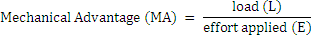Mechanical advantage has no unit. It is the simple ratio of two forces and affected by friction.

Velocity ratio

If a machine overcomes a load ‘L’ and the distance travelled by the load is ‘Ld’. Similarly, the effort applied in the machine is ‘E’ and the distance travelled by effort is ‘Ed’, then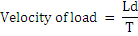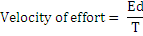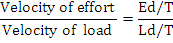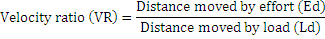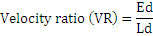Velocity ratio of simple machine is the ratio of distance travelled by an effort to the distance travelled by the load in the machine. As velocity ratio or ideal mechanical advantage is a simple ratio of two distances, it also does not have the unit. The friction is not involved in it.
Efficiency

If a machine overcomes a load ‘L’ and the distance travelled by the load is ‘Ld’, the work done by the load is L × Ld. It is also called output work or useful work. Therefore,

Output work = L × Ld

Likewise, the effort applied to overcome the load is E and the distance covered by effort is Ed, the work done by effort is E × Ed. It is also called input work. Therefore,

Input work = E × Ed

The efficiency of a simple machine is defined as the ratio of useful work done by machine (output work) to the total work put into the machine (input work).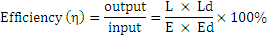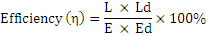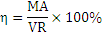For ideal or perfect machine, work output will be equal to the work input. Ideal machinesare those imaginary machines which are frictionless. In practice, the work output of a machine is always less than work input due to the effect of friction. If the frictional force in the machine increases the efficiency decreases. Because machines are frictionless in practice, the efficiency of a machine can never be 100%.
Relation between MA, VR, and η

We know that,

η=$$\frac{output \;work} {input\; work}$$ ×100%
or,η=$$\frac{work\;done\;by\;load} {work\;done\;by\;effort}$$×100%

or,η=$$\frac{L×Ld}{E×Ed}$$×100%

But the mechanical advantages =$$\frac{L}{E}$$ and velocity ratio = $$\frac{E_d}{L_d}$$

η=$$\frac{MA}{VR}$$ ×100%

Thus, the relation shows that efficiency is the ratio of mechanical advantage to velocity ratio in percentage.

#### Principle of simple machine

The principle of simple machine states that “if there is no friction in a simple machine, work output and work input are found equal in that machine”

Mathematically,

Output work = Input work

Or, L × Ld = E × Ed

• Those tools or devices which make our work easier and convenient in the direction of force is called simple machine.
• Simple machine helps us by magnifying force, accelerating work and by changing the direction of force.
• Velocity ratio of simple machine is the ratio of distance traveled by effort to the distance traveled by load in the machine.
• The efficiency of a simple machine is defined as the ratio of useful work done by machine (output work) to the total work put into machine (input work).
• The ratio of the load to the effort in a simple machine is called mechanical advantages or actual advantage of the machine.
.

#### Click on the questions below to reveal the answers

Simple machine is a device which is mechanically simple and helps to make our work easier and convenient. They are of six types:

1. Lever
2. Pulley
3. Wheel and axle
4. Inclined plane
5. Screw
6. Wedge

• By multiplying force
• By changing the direction of applied force
• By gaining speed to work faster
• By doing jobs which would be dangerous and unsafe.

It is defined as the ratio of load to the effort applied.
Mechanical advantage (M.A) =During calculation, friction is not counted but there is always friction inside a machine. So, actual value of MA is not same as the calculated value of it.

It is defined as the ratio of distance moved by the effort to the distance moved by the load .
Velocity ratio(VR) =It is because friction is not involved in VR.

Practically, no machine are frictionless. The friction decreases the mechanical advantage and efficiency of a machine whereas the VR of the machine is not affected by friction. So, value of MA is always less than value of VR in a simple machine.

The work done by the machine in overcoming the load is called output work.

The work done on the machine by the effort is called input work.
i.e. Input work = Effort x effort distance

The principle of simple machine states that if there is no friction in a simple machine, work output and work input are found to be equal in the machine.

The percentage ratio of the output work to the input work is called efficiency of a simple machine. Mathematically, η =x 100%

A machine having 100% efficiency is called an ideal or a perfect machine.

Efficiency of a simple machine is always less than 100% because friction reduces the input work applied on the machine.

Friction is the main factor which reduces the efficiency of a simple machine. Efficiency of a simple machine can be increased by reducing the friction. But oiling and greasing the machine is a useful way to increase the efficiency of a machine.

The energy input to a machine is greater than its energy output. Source energy is always wasted to overcome the friction and even to move the parts of the machine itself. Hence, no machine is perfect.

We know that, Efficiency(η) =x 100% or, η =x 100% or, η =x 100% or, η =x 100% Hence, proved.

It means that 70% of the input energy is obtained as useful energy. Rest of the 30% is used in overcoming friction.

The grease does not affect the VR of a machine as grease reduces friction and the VR of a machine is not affected by friction.

Load distance (Ld) = 10cm = 10/100m = 0.1m

Effort (E) = 100N
Effort distance (Ed) = 1m
• Mechanical advantage (MA) === 3
• Velocity ratio (VR) === 10
• Efficiency (η) =x 100% =x 100% = 30%

Effort (E) = 150N
Load distance (Ld) = 25cm = 0.25m
Effort distance (Ed) = 1- 0.25 = 0.75m
We know that, Efficiency (η) =x 100% =x 100% =x 100% = 100%

It is a crowbar used as first class lever of the simple machine.
Load distance (Ld) = 20 cm = 0.2m
Effort distance (E.d) = 70cm = 0.7m
Effort (E) = ?
Now, to balance it,
E x Ed = Ld
or, E x 0.7 = 0.2 x 10
or, E = 2/0.7
or, E = 20/7 N
Now,
Efficiency (η) =x 100% =x 100% = 100%

Suppose unknown weight (W) is load and 40N and 20N act as an effort
To balance lever,
E x E.d = L x L.d
Or, E1 x Ed1 + E2 x Ed2 = W x 25cm
Or, 40 x 5 + 20 (20 +5) = W x 25cm
Or,700 = W x 25
Or, 700/25 = W
Or, 28 = W

Hence, the required weight is 28N.

Given,
Effort (E) = 60N
It is a single movable pulley, so, VR = 2,
Efficiency (η) = ?
Now,
Mechanical advantage (M.A) === 3/2
And,
Efficiency (η) =x 100% =x 100% = 75%

Efficiency (η) = 90%
Effort (E) = 400N
Velocity ratio (VR) = 2
According to formula,
η =x 100% =x 100%
or , 90% =x 100%
or, L == 720N
Hence, 720 N can be lifted.

Effort (E) = 50N
Velocity Ratio (VR) = No. of pulley used = 4
Efficiency (η) = ?
We know,
η =x 100% =x 100%
=x 100% = 75%
Hence, the efficiency of the machine is 75%.

Given,
Velocity ratio (VR) = 4
Effort (E) = 36N
Total load (L) = 115 + 5N = 120N
MA == 120/36 = 3.33
and efficiency(η) =x 100% =x 100% = 83.25%
When load is raised by 5m,
And VR ==Or, 4 =∴E.d = 20m
So, work input = E x E.d = 36 x 20 = 720J
Work output = L x Ld = 120 x 5 = 600J
Total energy lost = Work input – Work output
= 720 – 600 = 120 J

Given,

Effort (E) = 16N
Effort distance (E.d) = length of slope = 3m
Load distance (L.d) = height of slope = 1.5m

Now,
MA == 24/16 = 3/2
VR == 3/1.5 = 2
Efficiency (η) =x 100% = ¾ x 100% = 75%

Given,
Pitch (P) = 1cm = 0.01m
Length of lever= 50cm = 0.5m
Effort (E) = ?
By using a condition,
MA = VR
or,=or,=or, E == 1.59 N
Now,
MA == 500/1.59 = 314.46
Hence, MA = 314.46 and E = 1.59N

Effort (E) = 100 N
Length of Lever (l) = 190 cm = $$\frac{190}{100}$$ = 1.9m
Effort DIstance (Ed) = length of lever - load distance = (1.9 - 0.2) = 1.7

Now,according to the formula ,
MA = $$\frac{L}{E}$$

= $$\frac{600}{100}$$
= 6

VR = $$\frac{ED}{LD}$$

= $$\frac{1.7 m}{0.20 m}$$ = 8.5

$$\eta$$= $$\frac{MA}{VR}$$ $$\times$$ 100%

= $$\frac{1.7m}{0.20}$$

= $$\frac{6}{8.5}$$$$\times$$ 100%

= 70.5 %

∴MA of the crowbar is 6, VR is 8.5 and $$\eta$$ is 70.5 %

Here, Load (L) = 600 N
Load distace (Ld) = 6 cm
Velocity ratio (VR) = 10
Efficiency $$\eta$$ = 80%

According to the formula ,

i. $$\eta$$ = $$\frac{MA}{VR}$$ $$\times$$ 100%

80% = $$\frac{MA}{10}$$ $$\times$$ 100%

or , 100 MA = 80 $$\times$$ 10

∴ MA = $$\frac{ 80 \times 10 }{100}$$ = 8

ii. MA = $$\frac{L}{E}$$

8 = $$\frac{600 N }{E}$$

or, E = $$\frac{600 N }{8}$$

= 75 N

iii. VR = $$\frac{Distance\;moved\;by\;the\;effort }{Distance\;moved\;by\;the\;load}$$

or, 10 = $$\frac{ ED }{6}$$

∴ Ed = 10 $$\times$$ 6 = 60cm = 0.6m

iv. Input work = E $$\times$$ Ed = 100 $$\times$$ 0.6m = 60J

v. Output Work = L $$\times$$ Ld = 600 $$\times$$ 0.06m = 36J

Hence, in this lever MA is 8 , effort applied is 75 N , distance moved by the effort moved by the effort is 60cm , input work is 60J and output work is 36J.

Here , Load (L) = 600 N
No.of pulleys = 5
Effort (E) = 300N
(i) MA = ?
(ii) VR = ?
(iii) Output Work = ?
(iv) Efficiency = ?

According to the formua ,

i. MA = $$\frac{L}{E}$$ = $$\frac{600N}{300N}$$ = $$\frac{60}{30}$$ = $$\frac{12}{6}$$

ii. VR = No.of pulleys used = 5

iii. Output Work = L $$\times$$ Ld = 600N $$\times$$ 4m = 2400J

For Effort Distance,
VR = $$\frac{Ed}{Ld}$$
5 = $$\frac{Ed}{4}$$
$$\therefore$$ Ed = 20m

iv. Input Work = E $$\times$$ Ed = 300N $$\times$$ 20m = 6000J

v. $$\eta$$ = $$\frac{Output\;work}{Input\;work}$$
= $$\frac{2400J}{6000J}$$
= $$\frac{24}{60}$$ $$\times$$ 100%
= 40%

Hence, MA is $$\frac{12}{6}$$ VR is 5 , work output is 2400J, work input is 6000J and $$\eta$$ is 40%

Here,
No.of pulleys = 4
i. E= ?
ii. VR=?
iii. $$\eta$$=?

According to the formula,
i. MA = $$\frac{L}{E}$$
or, 3 = $$\frac{400}{E}$$
or, E = $$\frac{400}{3}$$ = 133.33N

ii. VR = No.of pulleys used = 4

iii.$$\eta$$= $$\frac{MA}{VR}$$ $$\times$$ 100% = $$\frac{3}{4}$$ = 75%

Hence, effort applied in it is 133.33N , VR is 4 and $$\eta$$is 75%

Here,

Effort (E) = 500N
Length of slope (L) = 8m
Height of slope (h) = 5m

i. Output Work = L $$\times$$ Ld (h) = 700 $$\times$$ 5m = 3500J
ii. Input Work = E $$\times$$ Ed (l) = 500 $$\times$$ 8m = 4000J
iii. MA = $$\frac{L}{E}$$ = $$\frac{700}{500}$$ = 1.4
iv. VR = $$\frac{l}{h}$$ = $$\frac{8m}{5m}$$ = 1.6
v. $$\eta$$ = $$\frac{MA}{VR}$$$$\times$$ 100%

Here,
Radius of wheel (R) = 20 cm
Radius of axle (r) = 5 cm
Effort (E) = 200N

ii, Velocity Ratio(VR)= ?
iii. Efficiency $$\eta$$ = ?

Accrding to the formua
i. MA = $$\frac{L}{E}$$ = $$\frac{1000}{200}$$ = 5

ii. VR = $$\frac{R}{r}$$ = $$\frac{20}{5}$$ = 4

iii. $$\eta$$ = $$\frac{MA}{VR}$$ $$\times$$ 100%
= $$\frac{5}{4}$$ $$\times$$ 100% = 125%

Hence, in that wheel and axle MA is 5, VR is 4 and $$\eta$$ is 125%

Here,
First weight (L1) = 30N
Second weight (E1) = 20N
Third weight (E2) = 10N
Distance between L1 and fulcrum (Ld1) = 30cm
Distance between L2 and fulcrum (Ed1) = 5cm
Distance between E1 and fulcrum (Ed2) = 20cm

Now,
Anticlockwise moment = L1 $$\times$$ Ld1
= 30 $$\times$$ 30 = 900N

Clockwisw moment = E1 $$\times$$ Ed1 $$\times$$ E2 $$\times$$ Ed2
= 20 $$\times$$ 5 + 10 $$\times$$ 20
= 300N

iii. The lever will not be balanced, as the clockwise moment and anticlockwise moment are not equal.

iv. To balance the lever by changing the location of 10N weight, there should be -
= E1 $$\times$$ Ed1 + E2 $$\times$$ Ed2 = L1 $$\times$$ Ld2
= 20 $$\times$$ 5 + 10 $$\times$$ Ed2 = 30 $$\times$$ 30
= 100+10Ed2 = 900
= $$\frac{900-100}{10}$$ = $$\frac{800}{10}$$ = 80cm

Thus, the lever can be belanced by keeping the 10N weight at a distance of 80cm from the lever.

0%

lever
Scissors
pulley
Scale

heavy
mol
power
Newton

Friction
sound
heat
light
• ### Main advantage of simple machine is?

no need of electricity
can be used by all
very cheap
Magnifies effort
• ### Which is not a way to reduce friction?

streamlining
using correct combination of surface in contact
using ball bearing
making contact surface rough

Pitch
frequency
Bel
Decibel

80 dB
120 dB
140 dB
100 dB

## ASK ANY QUESTION ON Machine

Forum Time Replies Report
##### Roshan

principle of simple machine